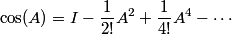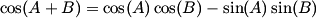# What is the cosine of a matrix?

How would you define the cosine of a matrix? If you’re trying to think of a triangle whose sides are matrices, you’re not going to get there. Think of power series. If a matrix A is square, you can stick it into the power series for cosine and call the sum the cosine of A.For example,This only works for square matrices. Otherwise the powers of A are not defined.

The power series converges and has many of the properties you’d expect. However, the usual trig identities may or may not apply. For example,only if the matrices A and B commute, i.e. AB = BA. To see why this is necessary, imagine trying to prove the sum identity above. You’d stick A+B into the power series and do some algebra to re-arrange terms to get the terms on the right side of the equation. Along the way you’ll encounter terms like A2 + AB + BA + B2 and you’d like to factor that into (A+B)2, but you can’t justify that unless A and B commute.

Is cosine still periodic in this context? Yes, in the sense that cos(A + 2πI) = cos(A). This is because the diagonal matrix 2πI commutes with every matrix A and so the sum identity above holds.

Why would you want to define the cosine of a matrix? One application of analytic functions of a matrix is solving systems of differential equations. Any linear system of ODEs, of any order, can be rewritten in the form x‘ = Ax where x is a vector of functions and A is a square matrix. Then the solution is x(t) = etA x(0). And cos(At) is a solution to x‘ ‘+ A2x = 0, just as in calculus.

## 13 thoughts on “What is the cosine of a matrix?”

1. Actually, (A+B)2 = A2 + AB + BA + B2 whether or not A commutes with B, although if they don’t commute this won’t equal A2 + 2AB + B2. The issue here is one of starting with (A+B)n, for all n, and being unable to group terms. Instead of getting a term for each combination of A and B, you get a term for each permutation and there’s just nothing sensible to be done with them that will bear any resemblance to the familiar world of commutative algebra.

2. human mathematics

Another application is in recommender systems. Cosine distance is one of the ways to compare my library to yours.

3. human mathematics: because inverse cosine is the angle between two unit vectors. See covariance and cosines.

4. Is the power series the best way to calculate cos(A) or are there other ways? In that case, which are they?

5. Is the power series the best way to calculate cos(A) or are there other ways? In that case, which are they? Jordanization?

6. Jordan canonical form is useful in exact hand calculations with small or special matrices. It’s unsuitable for numerical calculation because it is discontinuous: the tiniest change to a matrix can change a 0 to a 1 in the JCF.

I imagine power series could be practical, though not always a power series centered at 0. You probably want to start with a nearby matrix that is easy to exponentiate.

Finding the cosine of A is equivalent to solving a system of differential equations. It may be better numerically to solve the differential equations directly.

7. Nabueh

This is similar to finding exp(tA) where A is a matrix using the power series. Someone has successfully used the Cayley-Hamilton theorem to bypass diagonalization or some Jordan Canonical (so finding eigen-vectors can be avoided too) form to find the Exp / sine / cosine. The way to do it is to break the matrix into its Spectral Decomposition into N_i, P_i where P’s are projection matrices (so finding exp / cos/sin is easy) and N’s are nilpotent (so finding exp / cos /sin is really just a finite sum).

8. CogitoErgoCogitoSum

Why would we have defined the cosine of a matrix in this way in the first place? First of all, it only applies to square matrices; secondly, its a rather complicated approach to answering the question.

Why couldnt we have defined the cosine of a matrix as the matrix of the cosines of its components?

Not only is this much simpler but it applies to all size and shapes of matrices.

9. You could define it that way, but it would be less useful. It would no longer be the solution to the differential equation at the end of the post.

10. Power series of operators are interesting.

Letting D be the derivative operator, i.e. Df = f’, we can see that the Maclaurin series of a function is
f(x) = f(0) + x f'(0) + (1/2) x^2 f”(0) + …
= ( (1 + x D + (1/2) x^2 D^2 + …) f ) (0)
= { since x is just a number here, not an operator }
= ( (1 + x D + (1/2) (x D)^2 + …) f ) (0)
= ( e^(x D) f ) (0).

This is closely related to the e^(-i p x) from quantum mechanics.

11. I never heard before about Cos(A), with A as a matrix. However, I learned as a student (long ago, then …) the definition of Exp(A) , defined with the the same series “trick”. Such Matrices exponentials are used in some reliability calculations, I have heard.

12. Here’s the cosine of that matrix

>>> cos(Matrix([[1, 2], [3, 4]])).rewrite(exp).rewrite(cos).simplify()
Matrix([
[sqrt(33)*sin(5/2)*sin(sqrt(33)/2)/11 + cos(5/2)*cos(sqrt(33)/2), -4*sqrt(33)*sin(5/2)*sin(sqrt(33)/2)/33],
[ -2*sqrt(33)*sin(5/2)*sin(sqrt(33)/2)/11, -sqrt(33)*sin(5/2)*sin(sqrt(33)/2)/11 + cos(5/2)*cos(sqrt(33)/2)]])
>>> _.evalf()
Matrix([
[ 0.855423165077998, -0.110876381010749],
[-0.166314571516123, 0.689108593561875]])

13. @Per Persson: unfortunately what you write isn’t correct, e.g., x²D² is different from (xD)² = xDxD = xxD + xxDD (the D also “hits” the x). Check by applying your operator to f(x)=x : x²D²f(x) = 0, (xD)²f(x) = xDxDx = xDx = x.